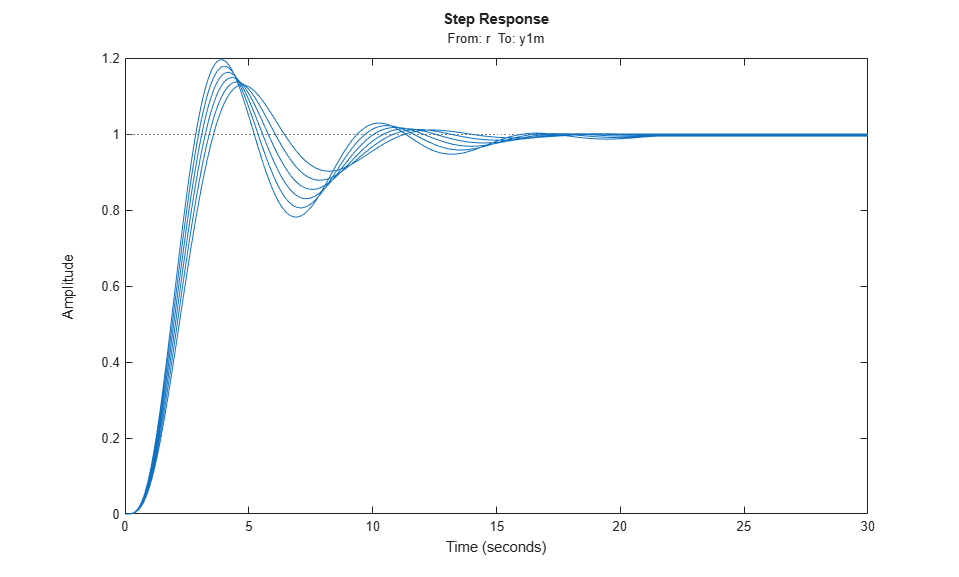Documentation

## Batch Linearize Model for Parameter Variations at Single Operating Point

In this example, you vary model parameters and linearize a model at its nominal operating conditions using the `linearize` command.

You can batch linearize a model for parameter variations at a single operating point to study:

• Plant dynamics for varying component tolerances.

• Controller robustness to variations in plant parameters.

• Transient responses for varying controller gains.

The `scdcascade` model contains two cascaded feedback control loops. Each loop includes a PI controller. The plant models, `G1` and `G2`, are LTI models.

For this model, the model operating point represents the nominal operating conditions of the system. Therefore, you do not have to trim the model before linearization. If your application includes parameter variations that affect the operating point of the model, you must first batch trim the model for the parameter variations. Then, you can linearize the model at the trimmed operating points. For more information, see Batch Linearize Model at Multiple Operating Points Derived from Parameter Variations.

To examine the effects of varying the outer-loop controller gains, linearize the model at the nominal operating point for each combination of gain values.

Open the model.

```sys = 'scdcascade'; open_system(sys) ```Define linearization input and output points for computing the closed-loop input/output response of the system.

```io(1) = linio('scdcascade/setpoint',1,'input'); io(2) = linio('scdcascade/Sum',1,'output'); ```

`io(1)`, the signal originating at the outport of the `setpoint` block, is the reference input. `io(2)`, the signal originating at the outport of the `Sum` block, is the system output.

To extract multiple open-loop and closed-loop transfer functions from the same model, batch linearize the system using an `slLinearizer` interface. For more information, see Vary Parameter Values and Obtain Multiple Transfer Functions.

Vary the outer-loop controller gains, `Kp1` and `Ki1`, within 20% of their nominal values.

```Kp1_range = linspace(Kp1*0.8,Kp1*1.2,6); Ki1_range = linspace(Ki1*0.8,Ki1*1.2,4); [Kp1_grid,Ki1_grid] = ndgrid(Kp1_range,Ki1_range); ```

Create a parameter structure with fields `Name` and `Value`. `Name` indicates which the variable to vary in the model workspace, the MATLAB® workspace, or a data dictionary.

```params(1).Name = 'Kp1'; params(1).Value = Kp1_grid; params(2).Name = 'Ki1'; params(2).Value = Ki1_grid; ```

`params` is a 6-by-4 parameter value grid, where each grid point corresponds to a unique combination of `Kp1` and `Ki1` values.

Obtain the closed-loop transfer function from the reference input to the plant output for the specified parameter values. If you do not specify an operating point, `linearize` uses the current model operating point.

```G = linearize(sys,io,params); ```

`G` is a 6-by-4 array of linearized models. Each entry in the array contains a linearization for the corresponding parameter combination in `params`. For example, `G(:,:,2,3)` corresponds to the linearization obtained by setting the values of the `Kp1` and `Ki1` parameters to `Kp1_grid(2,3)` and `Ki1_grid(2,3)`, respectively. The set of parameter values corresponding to each entry in the model array `G` is stored in the `SamplingGrid` property of `G`. For example, examine the corresponding parameter values for linearization `G(:,:,2,3)`:

```G(:,:,2,3).SamplingGrid ```
```ans = struct with fields: Kp1: 0.1386 Ki1: 0.0448 ```

To study the effects of the varying gain values, analyze the linearized models in `G`. For example, examine the step responses for all `Kp2` values and the third `Ki1` value.

```stepplot(G(:,:,:,3)) ```